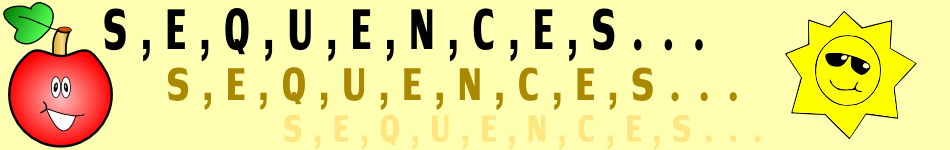There are 366 different Starters of The Day, many to choose from. You will find below some starters on the topic of Sequences. A lesson starter does not have to be on the same topic as the main part of the lesson or the topic of the previous lesson. It is often very useful to revise or explore other concepts by using a starter based on a totally different area of Mathematics.

Main Page

Sequences Starters:Add up a sequence of consecutive numbers. Can you find a quick way to do it?The numbers on five houses next to each other add up to 70. What are those five numbers?Find the missing terms from these linear sequences.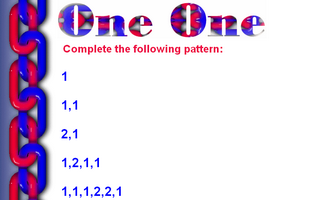Continue the given number pattern with the help of a little lateral thinking.A question which can be best answered by using algebra.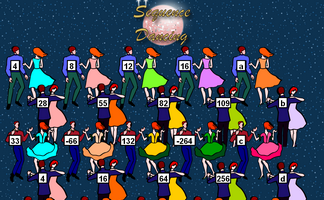Find the next term of the number sequences.Continue the sequences if you can work out the rule.Find the next term of the given number sequences. Can you also find a general rule for predicting the nth term of the sequence?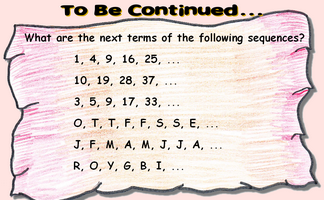Work out the next term in the given sequences.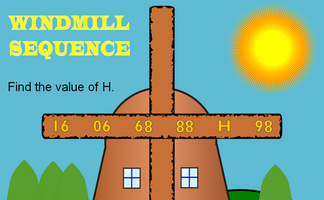Find the value of the missing term of the sequence. It is easier than you may think!

Exercises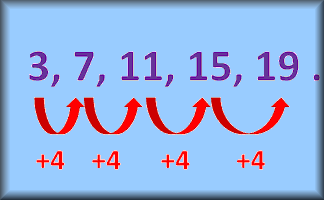Arithmetic Sequences

An exercise on linear sequences including finding an expression for the nth term and the sum of n terms.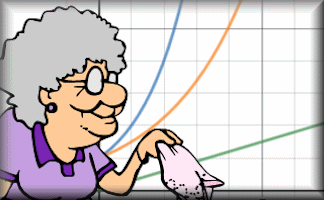Aunt Lucy's Legacy

Decide which of the four schemes Aunt Lucy proposes will provide the most money. This investigation involves the sum of sequences as well as considering life expectancy.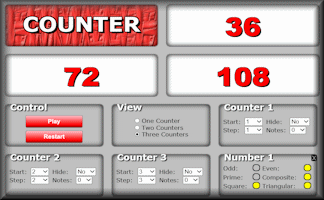Counter

A dynamic visual aid that counts! Choose the first term, common difference and the speed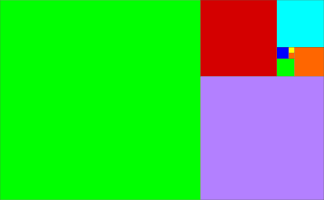Fibonacci Quest

A number of self marking quizzes based on the fascinating Fibonacci Sequence.Four Ever

Generate a number sequence based on the number of letters needed to spell the previous number.Geometric Sequences

An exercise on geometric sequences including finding the nth term and the sum of any number of terms.Interest

Practise using the formulas for simple interest and compound interest.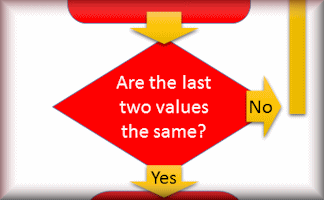Iteration

Find approximate solutions to equations numerically using iteration.Matchstick Patterns

Create a formula to describe the nth term of a sequence by examining the structure of the diagrams.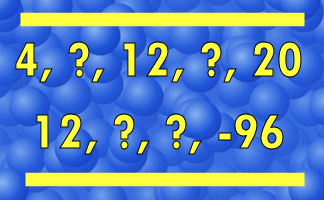Missing Terms

Can you work out which numbers are missing from these number sequences?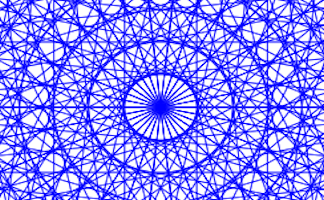Mystic Rose

Investigate the properties of the Mystic Rose by using this interactive diagram.Parts of Sequences

Find the formula that describes the part of the sequence that can be seen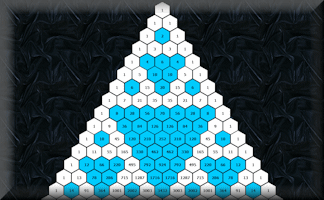Pascal's Triangle

Get to know this famous number pattern with some revealing learning activities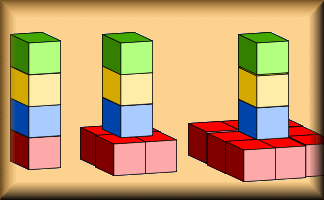Deduce expressions to calculate the nth term of quadratic and cubic sequences.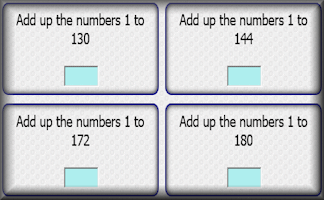Find the sum of a sequence of consecutive numbers using a quick, efficient, elegant method.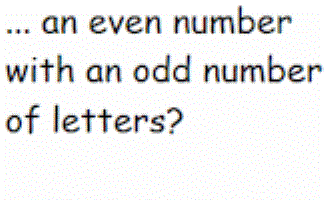Sequence Generator

An online app which produces number sequences as words.Sigma

Practise using the sigma notation to find the sum of various number series.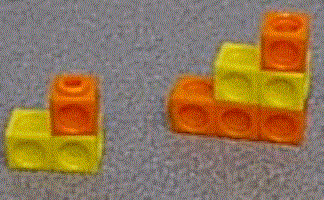Steps

Investigate the numbers associated with this growing sequence of steps made from Multilink cubes.Tower of Hanoi

Move the pieces of the tower from one place to another in the minimum number of moves.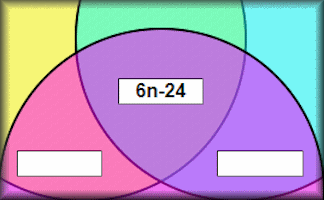Venn Diagram of Sequences

Find the formula for the nth term of sequences that belong in the given sets.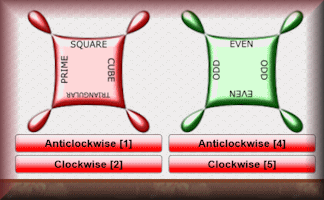Rotate the cogs to catch the flying numbers in the correct sections.

Search

The activity you are looking for may have been classified in a different way from the way you were expecting. You can search the whole of Transum Maths by using the box below.

Have today's Starter of the Day as your default homepage. Copy the URL below then select
Tools > Internet Options (Internet Explorer) then paste the URL into the homepage field.

Set as your homepage (if you are using Internet Explorer)

Do you have any comments? It is always useful to receive feedback and helps make this free resource even more useful for those learning Mathematics anywhere in the world. Click here to enter your comments.For All: